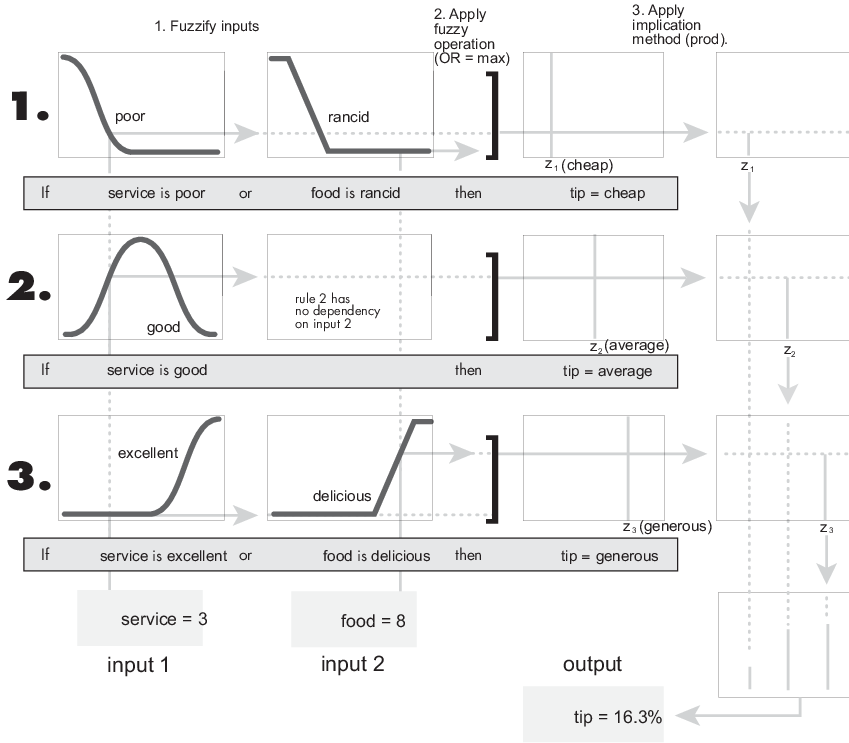Documentation

### This is machine translation

Mouseover text to see original. Click the button below to return to the English version of the page.

## What Is Sugeno-Type Fuzzy Inference?

This topic discusses the Sugeno, or Takagi-Sugeno-Kang, method of fuzzy inference. Introduced in 1985 , this method is similar to the Mamdani method in many respects. The first two parts of the fuzzy inference process, fuzzifying the inputs and applying the fuzzy operator, are the same. The main difference between Mamdani and Sugeno is that the Sugeno output membership functions are either linear or constant.

A typical rule in a Sugeno fuzzy model has the form:

 If Input 1 is x and Input 2 is y, then Output is z = ax + by + c

For a zero-order Sugeno model, the output level z is a constant (a = b = 0).

Each rule weights its output level, zi, by the firing strength of the rule, wi. For example, for an AND rule with Input 1 = x and Input 2 = y, the firing strength is

`${w}_{i}=AndMethod\left({F}_{1}\left(x\right),{F}_{2}\left(y\right)\right)$`

where F1,2(.) are the membership functions for Inputs 1 and 2.

The final output of the system is the weighted average of all rule outputs, computed as

where N is the number of rules.

A Sugeno rule operates as shown in the following diagram.### Note

Sugeno systems always use product implication and sum aggregation.

The preceding figure shows the fuzzy tipping model developed in Fuzzy Inference Process adapted for use as a Sugeno system. Fortunately, it is frequently the case that singleton output functions are sufficient for the needs of a given problem. As an example, the system `tippersg.fis` is the Sugeno-type representation of the now-familiar tipping model. If you load the system and plot its output surface, you see that it is almost the same as the Mamdani system you have previously seen.

```fis = readfis('tippersg'); gensurf(fis)```The easiest way to visualize first-order Sugeno systems is to think of each rule as defining the location of a moving singleton. That is, the singleton output spikes can move around in a linear fashion in the output space, depending on what the input is. This also tends to make the system notation compact and efficient. Higher-order Sugeno fuzzy models are possible, but they introduce significant complexity with little obvious merit. Sugeno fuzzy models whose output membership functions are greater than first order are not supported by Fuzzy Logic Toolbox™ software.

Because of the linear dependence of each rule on the input variables, the Sugeno method is ideal for acting as an interpolating supervisor of multiple linear controllers that are to be applied, respectively, to different operating conditions of a dynamic nonlinear system. For example, the performance of an aircraft may change dramatically with altitude and Mach number. Linear controllers, though easy to compute and suited to any given flight condition, must be updated regularly and smoothly to keep up with the changing state of the flight vehicle. A Sugeno fuzzy inference system is suited to the task of smoothly interpolating the linear gains that would be applied across the input space; it is a natural and efficient gain scheduler. Similarly, a Sugeno system is suited for modeling nonlinear systems by interpolating between multiple linear models.

To see a specific example of a system with linear output membership functions, consider the one-input, one-output system stored in `sugeno1.fis`. Load the system and view the properties of its output variable.

```fis = readfis('sugeno1'); fis.Outputs(1)```
```ans = fisvar with properties: Name: "output" Range: [0 1] MembershipFunctions: [1x2 fismf] ```

The output variable has two membership functions. View the properties of the first membership function.

`fis.Outputs(1).MembershipFunctions(1)`
```ans = fismf with properties: Name: "line1" Type: "linear" Parameters: [-1 -1] ```

View the properties of the second membership function.

`fis.Outputs(1).MembershipFunctions(2)`
```ans = fismf with properties: Name: "line2" Type: "linear" Parameters: [1 -1] ```

Further, these membership functions are linear functions of the input variable. The membership function `line1` is defined by the equation:

`$output=\left(-1\right)×input+\left(-1\right)$`

and the membership function `line2` is:

`$output=\left(1\right)×input+\left(-1\right)$`

The input membership functions and rules define which of these output functions are expressed and when:

`fis.Rules`
```ans = 1x2 fisrule array with properties: Description Antecedent Consequent Weight Connection Details: Description _________________________________ 1 "input==low => output=line1 (1)" 2 "input==high => output=line2 (1)" ```

The function `plotmf` shows us that the membership function `low` generally refers to input values less than zero, while `high` refers to values greater than zero. The function `gensurf` shows how the overall fuzzy system output switches smoothly from the line called `line1` to the line called `line2`.

```subplot(2,1,1) plotmf(fis,'input',1) subplot(2,1,2) gensurf(fis)```As this example shows, Sugeno-type system gives you the freedom to incorporate linear systems into your fuzzy systems. By extension, you could build a fuzzy system that switches between several optimal linear controllers as a highly nonlinear system moves around in its operating space.

 Sugeno, M., Industrial applications of fuzzy control, Elsevier Science Pub. Co., 1985.